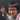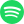# Discrete Calculus: Linearity

July 27, 2020 · 23:42 pm · Discrete CalculusMath

For an operator to be a linear function, they must meet two criterias:

2. Homogeneity of degree 1, i.e. scalar multiplication is preserved

In other words, the following are satisfied:

• $f(x+y) = f(x) + f(y)$
• $f(cx) = c f(x)$

for the function $f$ of interest. It turns out that discrete derivatives satify these conditions.

\begin{aligned} \Delta_h[f + g](n) &= \frac{[f+g](n + h) - [f+g](n)}{h} \\ &= \frac{f(n + h) + g(n + h) - (f(n) + g(n))}{h} \\ &= \frac{f(n+h)-f(n)}{h} + \frac{g(n+h)-g(n)}{h} \\ &= \Delta_h f(n) + \Delta_h g(n) \space \blacksquare \\ \end{aligned}

## Homogeneity of degree 1

\begin{aligned} \Delta_h[cf](n) &= \frac{[cf](n + h) - [cf](n)}{h} \\ &= \frac{c f(n+h) - c f(n)}{h} \\ &= c \cdot \frac{f(n+h) - f(n)}{h} \\ &= c \Delta_h f(n) \space \blacksquare \\ \end{aligned}

So we can conclude that the discrete derivative as an operation is a linear transformation in the function space.Streaming direct thought dumps from Yuto Nishida.
Connect with me on LinkedIn! I'm currently listening to: